# Difference in Math

Subtraction is one of the most important math skills you’ll ever learn. Discover all of the different ways to find the difference.Author
Taylor HartleyExpert Reviewer

Published: August 24, 2023# Difference in Math

Subtraction is one of the most important math skills you’ll ever learn. Discover all of the different ways to find the difference.Author
Taylor HartleyExpert Reviewer

Published: August 24, 2023# Difference in Math

Subtraction is one of the most important math skills you’ll ever learn. Discover all of the different ways to find the difference.Author
Taylor HartleyExpert Reviewer

Published: August 24, 2023Key takeaways

• Finding the difference is foundational – Subtraction is one of the most basic concepts in math, so it’s important to master the skill before moving on.
• There are various ways to find the difference – Someone might ask you to find the difference using a number line, but for more complex subtraction problems, you might have to use a method called borrowing.
• Practice makes perfect – Once you know how to find the difference, keep practicing to increase your speed and accuracy!

Finding the difference, or subtracting, is one of the most important yet basic concepts in math. Without this skill, it will be almost impossible to move on to more complicated math concepts like multiplying and dividing. Plus, you’ll want to master the basics of subtraction before you venture into subtracting fractions and decimals — heads up 4th and 5th graders! Let’s dig in to learn more about the difference in math.

## What is the difference in math?If you have already mastered finding the sum, learning how to find the difference in math will be a breeze! The difference in math is what you get when you subtract two numbers. Basically, it just tells us how much one number differs from another number — and it’s an important skill to practice. Before we dive in, let’s cover a few key elements that will help us find the difference.

The math symbol for the difference is expressed as a minus sign (-). If you see an equation like this: 10 – 2 = 8, the 10 is called the minuend, the 2 is called the subtrahend, and the 8 is called the difference.

## How to find the difference between two numbersThere are plenty of ways to find the difference in math. Finding the method that works best for you is key! Some people are visual learners and prefer to use a number line, while others like to stack the equation vertically and use the borrowing method — both get you to the same place!

Let’s explore the different ways that you can find the difference between two numbers.

### Finding the difference using a number lineOne way to find the difference in math is to use a number line — it’s a great visual aid and very easy to learn. For this method, locate the minuend, or the first number in the equation, on the number line and move left on the number line until you land on the subtrahend, or the second number in the equation. While you’re moving left, simply count the amount of hops you made to get to the subtrahend.

### Finding the difference with borrowingThe first step to finding the difference with borrowing is to place the values, or numbers, vertically in the order of their place values. You’ll also want to make sure that the greater number is on top and the smaller number is on the bottom. Next, you’ll start subtracting the numbers, starting from the ones place.

If you’re asked to find the difference between 7,869 and 4,684, your equation when using the borrowing method should be set up like this: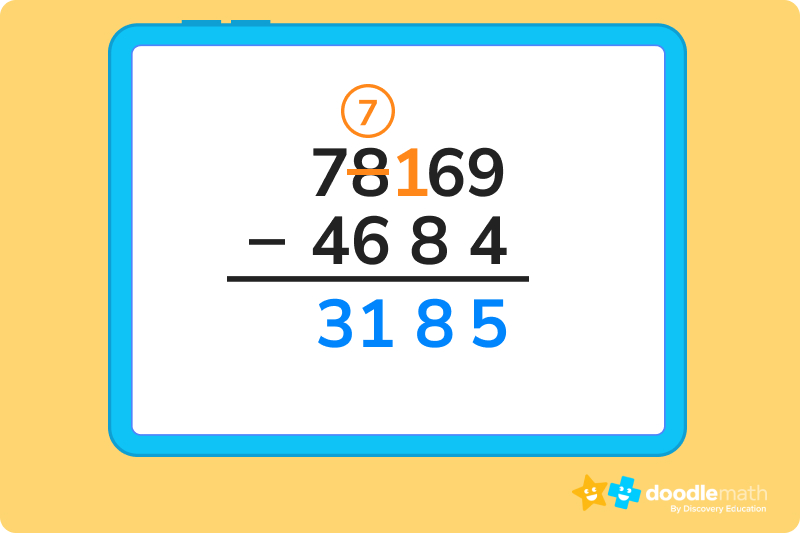In this case, some digits in the subtrahend are greater at a certain place value than the corresponding number in the minuend — but that’s okay! To find the difference, start by subtracting 4 from 9 to get 5. The subtrahend was not greater than the minuend in this place value, so you can move on.

Next, find the difference between 6 and 8. Here, the subtrahend is bigger than the minuend, so you’ll need to borrow! Borrow 1 from the digit that is at the 100s place in the minuend, making it a 7 instead of an 8. Now, the number in the 10s place, the 6, becomes a 16. You can now easily find the difference between 16 and 8 which is 8!

Now, move to the 100s place and find the difference between our new 7 and 6. The difference is just 1. Lastly, find the difference between 7 and 4. The answer is 3. The difference between 7,869 and 4,684 is 3,185. If there are multiple minuend digits that are greater than their corresponding subtrahend digits, simply repeat the borrowing process, always taking one number from the digit to the left and adding a 1 in front of the minuend number you’re working with. It’s important to note that you’re not adding 1, but putting the number 1 in front of the minuend digit. So in this example, the 6 became a 16, not a 7.

### Finding the difference without borrowingAgain here, the first step to finding the difference without borrowing is to place the values, or numbers, vertically in the order of their place values. You’ll also want to make sure that the first number is on top and the number you are subtracting is on the bottom of the equation. Next, you’ll start subtracting the numbers, starting from the ones place.

If you’re asked to find the difference between 7,869 and 3,653, your equation when you’re not borrowing should be set up like this: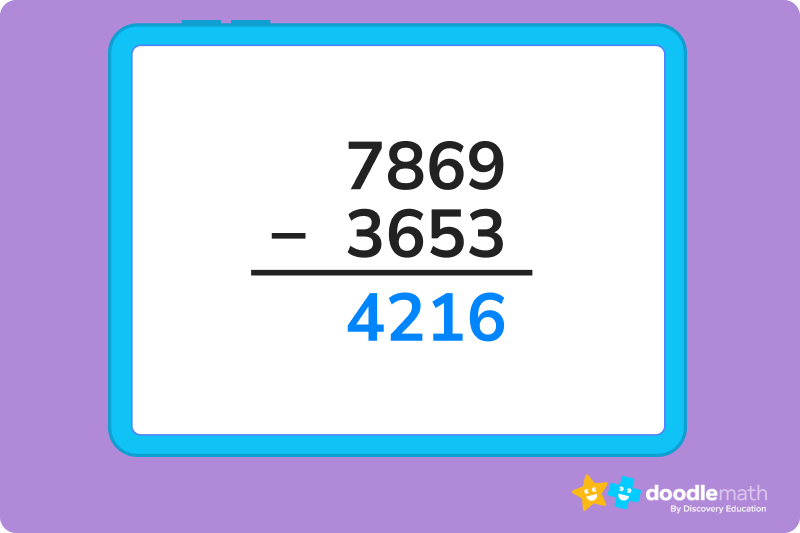We can see that each digit in the subtrahend is less than its corresponding digit in the minuend, so we can do simple subtraction to find the difference! The difference between 9 and 3 is 6, between 6 and 5 is 1, between 8 and 6 is 2, and between 7 and 3 is 4. So, the difference between 7,869 and 3,653 is 4,216.

## More advanced ways to work with differenceYou might have already guessed it, but there are so many more advanced applications and methods to finding the difference in math. For now, we’ll give brief examples of how to find the difference between two fractions and decimals to get you familiar, but you probably won’t need to use these skills until 4th and 5th grade!

### Finding the difference between two fractionsTo find the difference between two fractions, there are a few steps you have to follow:

• Check to see if the fractions are like fractions. If they are, you can skip to bullet number 4. If they’re not like fractions, move to the second bullet.
• When the fractions are unlike, we need to find the least common denominator, or LCM. We’ll include an example below the steps!
• Convert each fraction into an equivalent fraction, or like fraction, having the denominator equal to the LCM found in the last step.
• After both fractions are like fractions, subtract their numerators, or the number on top.
• Finally, reduce the fraction to the simplest form if needed.

Phew — okay, now it’s time for an example! If a recipe calls for ⅓ sugar and 2⁄7 milk, how much more sugar is there compared to milk?

First, we know that the fraction that symbolizes sugar = 1⁄3

Second, we know that the fraction that symbolizes milk = 2⁄7

The LCM of 3 and 7 is 21.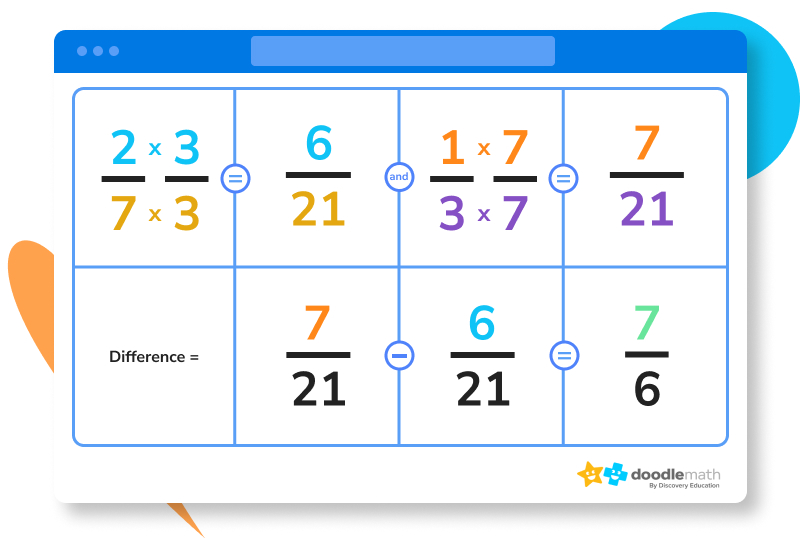### Finding the difference between two decimalsTo find the difference between two decimal numbers, there are a few steps that you have to follow:

• Convert unlike decimals into like decimals.
• Place the decimal numbers so that the tens digit is below the other tens digit, the ones below the ones, the decimal point below the decimal point, and so on. This will look similar to the equation example above when we practiced borrowing!
• Subtract in the same way we do for whole numbers, and use your borrowing skills when you have to.
• Place the decimal point in the difference, or the answer, exactly below the decimal point in the original decimal numbers of the minuend and subtrahend.

Ready for another quick example? And don’t worry if it’s a bit confusing to start — again, this will be more thoroughly explained in 4th and 5th grade!

Let’s find the difference between 39.03 and 8.762.

We will first need to convert both the decimal numbers into like decimals by adding one zero in 39.03. So, it can now be written as 39.030.

Next, subtract them like whole numbers, borrowing when needed, and place a decimal point. The difference is 30.268.

## Let’s practice together!Need to run through a few more problems on your own? Keep practicing!

1. Find the difference between 9 and 5 on a number line. (Answer: 4)
2. What’s the difference between 926 and 658? (Answer: 268)
3. Find the difference between 764 and 342. (Answer: 422)

Ready to give it a go?

Now that we know how to find the difference using multiple different methods, we’re ready to do a few more practice problems to put these new skills to the test! Work through the following problems on your own. Feel free to look back at the practice problems above if you get stuck, or if you need a quick reminder on how to find the difference.

And remember, don’t get discouraged if you run into a roadblock! Practice is the best way to learn something new.

## Parent Guide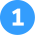How did we get here?

1. Read the problem: Using a number line, find the difference between 8 – 3.
2. Using a number line, locate the 8 and place your pencil on the line.
3. Then, make small loops along the way as you hop to the left until you get to the number 3.
4. There are 5 hops between 8 and 3 on the number line.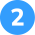B is incorrect.

How did we get here?

1. Read the problem: Which of the following is incorrect?
1. 5 – 2 = 3
2. 18 – 13 = 6
3. 726 – 653 = 73
4. 281 – 160 = 121
2. For each problem, find the difference between the two numbers. You may use a number line, or you may have to borrow.
3. The answer to 5 – 2 = 3 which is correct.
4. The answer to 18 – 13 = 5. The equation above shows 18 – 13 = 6 which is incorrect.
5. The answer to 726 – 653 = 73 which is correct.
6. The answer to 281 – 160 = 121 which is correct.
7. Based on the answers you found to each equation, find the one that was incorrect.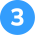How did we get here?

1. Read the problem: Fill in the blank: 24 – __ = 18
2. Using a number line, locate the 24 and place your pencil on the line.
3. Move your pencil to the left until you reach the 18, counting how many spaces are in between 24 and 18.
4. There are 6 spaces, or hops, on the number line between 24 and 18.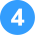How did we get here?

1. Read the problem: What is the difference between 925 and 568?
2. Rewrite the equation vertically with the larger greater on top, lining up all of the place values.
3. Use the borrowing method twice to find the difference between 5 and 8 and again to find the difference between 2 and 6.
4. The difference between 925 – 568 is 357.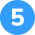How did we get here?

1. Read the problem: Sam has 23 cakes, and Keith has 5. What is the difference?
2. Using a number line, locate the 23 and place your pencil on the line.
3. Move your pencil to the left 5 hops.
4. Your pencil will land on the 18.
5. The difference between 23 – 5 = 18.

## FAQs about math strategies for kidsWe understand that diving into new information can sometimes be overwhelming, and questions often arise. That’s why we’ve meticulously crafted these FAQs, based on real questions from students and parents. We’ve got you covered!

In math, the term “difference” refers to the result of subtracting one number from another. It indicates the numerical gap or distance between two quantities. On the other hand, “sum” refers to the result of adding two or more numbers together.

To find the difference between two numbers, subtract the smaller number from the larger number. The result will be the numerical gap or difference between the two values.

Regrouping, also known as borrowing or renaming, is a technique used in subtraction when the digit being subtracted from is smaller than the digit being subtracted. It allows us to perform the necessary subtraction by “borrowing” or regrouping from the next higher place value.

Subtraction is going to be absolutely crucial as you climb up the mountain of mathematical knowledge! It provides us with a way to calculate differences, solve problems, analyze data, establish relationships, and work with algebraic expressions.## Related Posts

Title 1

Title 2

Title 3

Lesson credits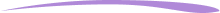Taylor Hartley

Taylor Hartley is an author and an English teacher based in Charlotte, North Carolina. When she's not writing, you can find her on the rowing machine or lost in a good novel.

Jill Padfield has 7 years of experience teaching high school mathematics, ranging from Alegra 1 to AP Calculas. She is currently working as a Business Analyst, working to improve services for Veterans while earning a masters degree in business administration.

Taylor Hartley

Taylor Hartley is an author and an English teacher based in Charlotte, North Carolina. When she's not writing, you can find her on the rowing machine or lost in a good novel.

Jill Padfield has 7 years of experience teaching high school mathematics, ranging from Alegra 1 to AP Calculas. She is currently working as a Business Analyst, working to improve services for Veterans while earning a masters degree in business administration.# Are you a parent, teacher or student?

Are you a parent or teacher?

## Hi there!

Book a chat with our team